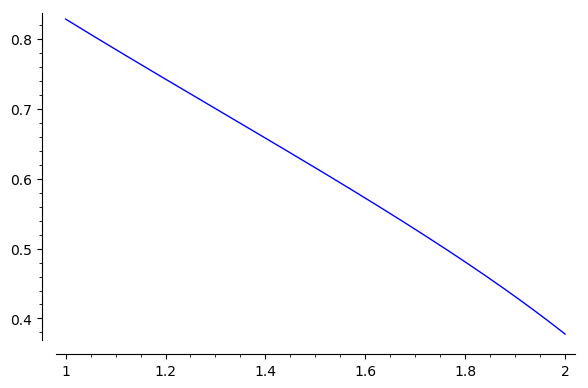# Numerical integration and plot failing

I have a function which can be efficiently calculated on some interval on the real line and if I feed Sage with concrete arguments, Sage gives concrete numerical values. Nevertheless, if I try to integrate it numerically (or plot it), something goes terribly wrong and Sage is not able to calculate the values.

In my case I get the error message

negative number cannot be raised to a fractional power


The function itself is a solution to the cubic equation so it involves square root and a cubic root.

is it some bug in sage and if there is some workaround?

MINIMAL NON_WORKING EXAMPLE

y = var('y')
z = var('z');

cauchy = y.substitute(solve(z*y^3 +y^2 - 2*z*y+2, y))

def F(u):
return arg(cauchy).substitute(z=u).n()

numerical_integral(F, 1, 2)

edit retag close merge delete

Indeed :

sage: var("y,z")
(y, z)
sage: cauchy(z)=solve(z*y^3 +y^2 - 2*z*y+2 ,y).rhs()
sage: def foo(u):return(arg(cauchy(u)).n())
sage: [foo(t) for t in (1,1.1..2)]
[0.828222717321238,
## Snip...
0.377665318352514]


Therefore, this function can be evaluated. But :

sage: plot(foo,(1,2))
verbose 0 (3635: plot.py, generate_plot_points) WARNING: When plotting, failed to evaluate function at 200 points.
verbose 0 (3635: plot.py, generate_plot_points) Last error message: 'negative number cannot be raised to a fractional power'
Launched png viewer for Graphics object consisting of 0 graphics primitives


... not in plot. Trying to plot symbolically yelds :

TypeError: Cannot evaluate symbolic expression to a numeric value.


A bug, IMHO

Sort by » oldest newest most voted

Here’s a workaround for plotting:

list_plot( [[t, F(t)] for t in (1,1.1..2)], plotjoined=True )


with a live example. For integration you could likewise evaluate the function at discrete points, add all interior evaluations plus half of the values at the endpoints and multiply by the step size (i.e., a discrete integral).

moreA different approach that works even in a Python 2 based Sage:

sage: var("y,z");
sage: cauchy(z) = y.subs(solve(z*y^3 + y^2 - 2*z*y + 2, y))
sage: F(z) = arctan2(imag(cauchy(z)),real(cauchy(z)))
sage: numerical_integral(F, 1, 2)
(0.6116545934235456, 6.790730127365591e-15)
sage: plot(F(z),(z,1,2))The plot is really slow. It may take more than 20 seconds. See this SageMath cell.

more

NOTE : This has replaced, (not complemented) my original answer concluding that this was a (Python) bug. Apologies for the confusion...

As pointed out by Serge Lelièvre, this seems a limitation of Python 2 floats, which does not exist in Python 3, where one can do :

sage: def F(u): return arg((u-3)^(1/3))
sage: F(1)
arg((-2)^(1/3))
sage: F(1).n()
1.04719755119660
sage: F(2).n()
1.04719755119660
sage: plot(F,(1,2))
Launched png viewer for Graphics object consisting of 1 graphics primitive
sage: integrate(F(x),x,1,2)
1/3*pi


See this sage-devel thread for further illumination...

In the original example:

sage: cauchy(z)=y.substitute(solve(z*y^3 +y^2 - 2*z*y+2, y))
sage: def G(u): return arg(cauchy(u))
sage: numerical_integral(lambda u:G(u), 1, 2)
(0.6116545934235456, 6.790730127365591e-15)

more

This works for me in (Python 3 based) Sage 8.8.beta7:

sage: y, z = var('y z')
sage: cauchy = y.substitute(solve(z*y^3 + y^2 - 2*z*y + 2, y))
sage: def F(u):
....:     return arg(cauchy).substitute(z=u).n()
....:
sage: numerical_integral(F, 1, 2)
(0.6116545934235456, 6.790730127365591e-15)


Here are links to Jupyter notebooks on CoCalc showing

This also fails with Py2-based SageMath 8.8.beta7.

So the "easy" solution for you is: switch to Python3-based Sage!

For this, follow the instructions to install from source, paying attention to the discussion about Python 3.

Or if you want to turn your existing SageMath into a Python3-based one, you could open a terminal, cd to your Sage directory, and type

make distclean
make configure
./configure --with-python=3
make


which will work if you have all the prerequisites.

more